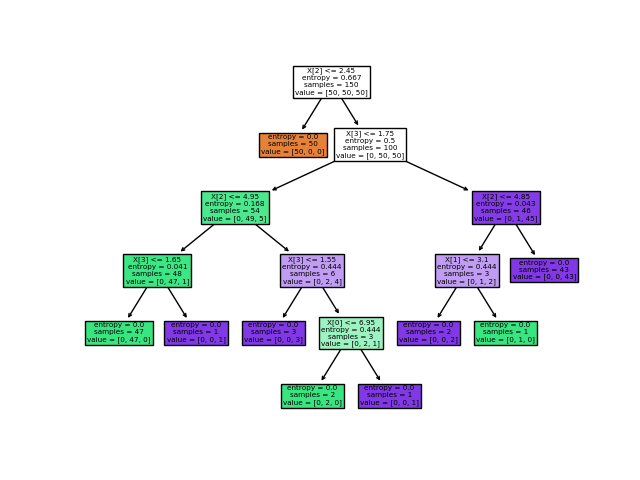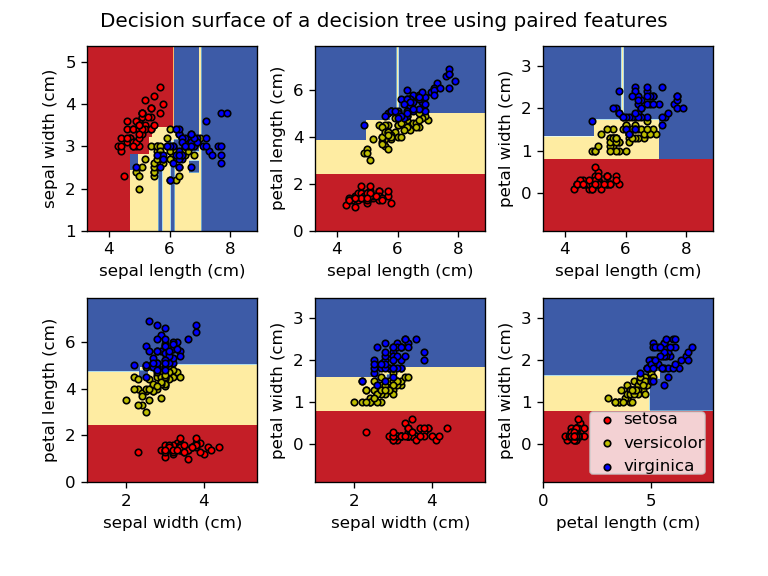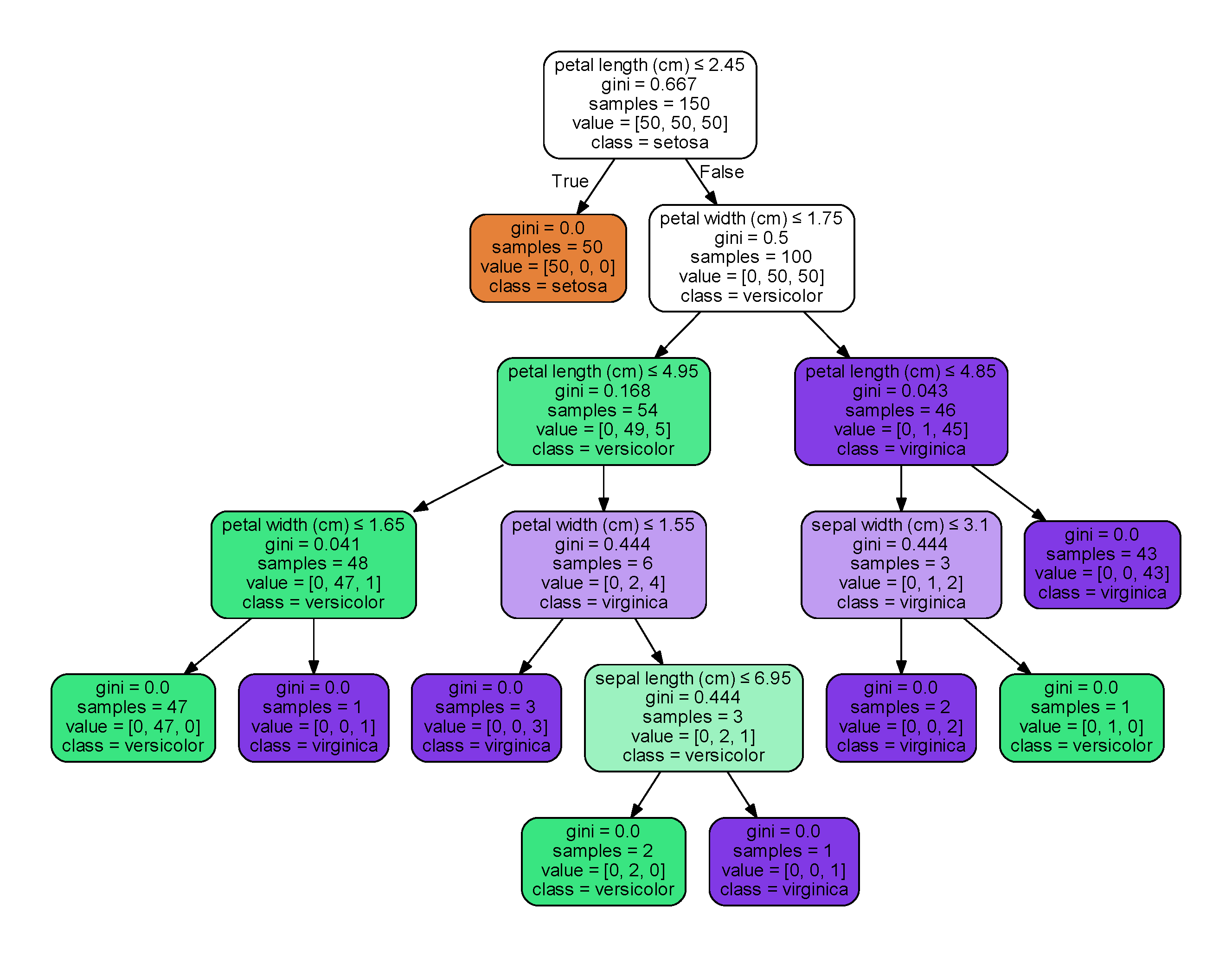# 大家的人工智能——决策树

### 决策树结构### 信息增益

$Ent(D) = - \sum_{k=1}^yp_klog_2p_k$
Ent(D)的值越小，那么D的纯度越高。

$Gain(D, a)=Ent(D) - \sum_{v=1}^V \frac{|D^v|}{|D|} Ent(D^v)$

### 增益率

$Gain\_ratio(D, a) = \frac{Gain(D, a)}{IV(a)}$

$IV(a) = -\sum_{v=1}^V\frac{|D^v|}{|D|}log_2 \frac{|D^v|}{|D|}$

IV(a)成为属性a的固有值（intrinsic value），属性a的可能取值越多，那么IV(a)越大。

### 基尼指数

$Gini(D) = \sum_{k=1}^y \sum_{k' \not=k} p_kp_{k'} = 1 - \sum_{k=1}^yp_k^2$

Gini(D)反应了从数据集中随机抽取两个样本，它们属于不同类的概率，因此Gini(D)越小，那么数据集D的纯度越高，那么属性a的基尼指数为：
$Gini\_index(D, a) = \sum_{v=1}^V \frac{|D^v|}{|D|}Gini(d^v)$

### 实践一下

for pairidx, pair in enumerate([[0, 1], [0, 2], [0, 3],
[1, 2], [1, 3], [2, 3]]):
# We only take the two corresponding features
X = iris.data[:, pair]
y = iris.target

# Train
clf = DecisionTreeClassifier().fit(X, y)

# Plot the decision boundary
plt.subplot(2, 3, pairidx + 1)

x_min, x_max = X[:, 0].min() - 1, X[:, 0].max() + 1
y_min, y_max = X[:, 1].min() - 1, X[:, 1].max() + 1
xx, yy = np.meshgrid(np.arange(x_min, x_max, plot_step),
np.arange(y_min, y_max, plot_step))

Z = clf.predict(np.c_[xx.ravel(), yy.ravel()])
Z = Z.reshape(xx.shape)
cs = plt.contourf(xx, yy, Z, cmap=plt.cm.RdYlBu)

plt.xlabel(iris.feature_names[pair])
plt.ylabel(iris.feature_names[pair])

# Plot the training points
for i, color in zip(range(n_classes), plot_colors):
idx = np.where(y == i)
plt.scatter(X[idx, 0], X[idx, 1], c=color, label=iris.target_names[i],
cmap=plt.cm.RdYlBu, edgecolor='black', s=15)clf = DecisionTreeClassifier().fit(iris.data, iris.target)
plot_tree(clf, filled=True)dot_data = export_graphviz(clf, out_file=None, feature_names=iris.feature_names, class_names=iris.target_names,
filled=True, rounded=True, special_characters=True)
graph = graphviz.Source(dot_data)
graph.render('iris')